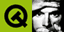DOC HOME SITE MAP MAN PAGES GNU INFO SEARCH PRINT BOOK
 Home | All Classes | Main Classes | Annotated | Grouped Classes | Functions# QMutex Class Reference

The QMutex class provides access serialization between threads. More...

All the functions in this class are thread-safe when Qt is built with thread support.

#include <qmutex.h>

## Detailed Description

The QMutex class provides access serialization between threads.

The purpose of a QMutex is to protect an object, data structure or section of code so that only one thread can access it at a time (This is similar to the Java synchronized keyword). For example, say there is a method which prints a message to the user on two lines:

```    int number = 6;

void method1()
{
number *= 5;
number /= 4;
}

void method2()
{
number *= 3;
number /= 2;
}
```

If these two methods are called in succession, the following happens:

```    // method1()
number *= 5;        // number is now 30
number /= 4;        // number is now 7

// method2()
number *= 3;        // nubmer is now 21
number /= 2;        // number is now 10
```

If these two methods are called simultaneously from two threads then the following sequence could result:

```    // Thread 1 calls method1()
number *= 5;        // number is now 30

//
// Most likely Thread 1 has been put to sleep by the operating
// system to allow Thread 2 to run.
number *= 3;        // number is now 90
number /= 2;        // number is now 45

number /= 4;        // number is now 11, instead of 10
```

If we add a mutex, we should get the result we want:

```    QMutex mutex;
int number = 6;

void method1()
{
mutex.lock();
number *= 5;
number /= 4;
mutex.unlock();
}

void method2()
{
mutex.lock();
number *= 3;
number /= 2;
mutex.unlock();
}
```

Then only one thread can modify number at any given time and the result is correct. This is a trivial example, of course, but applies to any other case where things need to happen in a particular sequence.

When you call lock() in a thread, other threads that try to call lock() in the same place will block until the thread that got the lock calls unlock(). A non-blocking alternative to lock() is tryLock().

## Member Function Documentation

### QMutex::QMutex ( bool recursive = FALSE )

Constructs a new mutex. The mutex is created in an unlocked state. A recursive mutex is created if recursive is TRUE; a normal mutex is created if recursive is FALSE (the default). With a recursive mutex, a thread can lock the same mutex multiple times and it will not be unlocked until a corresponding number of unlock() calls have been made.

### QMutex::~QMutex () [virtual]

Destroys the mutex.

Warning: If you destroy a mutex that still holds a lock the resultant behavior is undefined.

### void QMutex::lock ()

Attempt to lock the mutex. If another thread has locked the mutex then this call will block until that thread has unlocked it.

### bool QMutex::locked ()

Returns TRUE if the mutex is locked by another thread; otherwise returns FALSE.

Warning: Due to differing implementations of recursive mutexes on various platforms, calling this function from the same thread that previously locked the mutex will return undefined results.

### bool QMutex::tryLock ()

Attempt to lock the mutex. If the lock was obtained, this function returns TRUE. If another thread has locked the mutex, this function returns FALSE, instead of waiting for the mutex to become available, i.e. it does not block.

If the lock was obtained, the mutex must be unlocked with unlock() before another thread can successfully lock it.